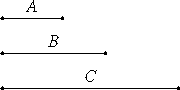# Proposition 28

If an odd number is multiplied by an even number, then the product is even.

Let the odd number A multiplied by the even number B make C.I say that C is even.

VII.Def.15

Since A multiplied by B makes C, therefore C is made up of as many numbers equal to B as there are units in A. And B is even, therefore C is made up of even numbers.

IX.21

But, if as many even numbers as we please be added together, the whole is even. Therefore C is even.

Therefore, if an odd number is multiplied by an even number, then the product is even.

Q.E.D.

## Guide

This is one of two propositions that examine the result of multiplying even and odd numbers by each other. The third proposition, the product of two even numbers, is omitted.

Note that the proof for this theorem makes no use of the assumption that A is an odd number. The statement of this theorem might just as well be “if any number is multiplied by an even number, then the product is even.”

#### Use of this proposition

The proof of proposition IX.31 concludes at one point that since a number divides a odd number, it must be odd. Such a statement follows from this proposition.